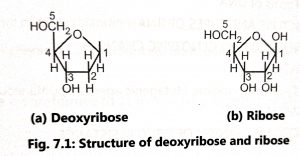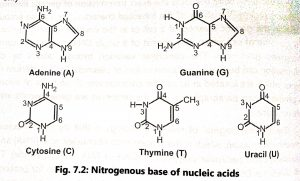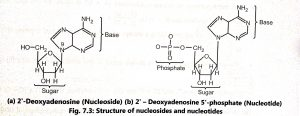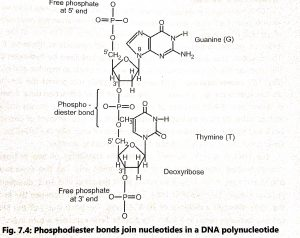# Chemical Nature of DNA

Chemical Nature of DNA

Deoxyribonucleic acid (DNA) and Ribonucleic acid (RNA) are the principal genetic materials of living organisms. They are chemically called nucleic acids. The DNA molecule (nucleic acid) is also called polynucleotide. It is a polymer and consists of a long chain of monomers called nucleotides. Each nucleotide consists of three main parts such as cyclic five-carbon sugar, purine or pyrimidine base and a phosphate group. The nucleic acids a classified into two types, based on the types of sugars such as ribose nucleic acid (RNA) and deoxyribose nucleic acid (DNA). The sugar present in DNA is a five-carbon pentose called 2’deoxyribose in which the -OH group on carbon 2 of ribose is replaced by hydrogen.Nucleotides contain one of four bases (Fig. 7.2) as adenine (A), guanine (G), thymine (T) or cytosine (C), Adenine and guanine contain two carbon-nitrogen rings (purines) and cytosine and thymine contain a single ring (pyrimidines). Uracil (U) is the third pyrimidine and it is found only in RNA.Nucleotides contain phosphate groups (PO4) attached to the 5′ carbon of the sugar. The nitrogenous bases combined with pentose sugar to form nucleosides and a nucleoside linked with phosphate form a nucleotide (Fig. 7.3).In DNA, a phosphate group (PO) is attached to the 3′-carbon of deoxyribose sugar and 5′-carbon of another sugar. Strong negative charges of nucleic acid are due to the presence of phosphate groups. The 5′ phosphate of one nucleotide forms a bond with the 3′ carbon of the next nucleotide eliminating the -OH group on the 3′ carbon during the reaction. The bond is called a 3′-5′ phosphodiester bond (Fig. 7.4).A chemist Erwin Chargaff (1950) established a number of important relationships concerning the contents of individual bases in DNA. Chargaff’s equivalence rule has been found to apply almost universally in different organisms. Chargaff’s rules are as follows:

• The total number of purine nucleotides (A + G) is equal to the total number of pyrimidine nucleotides (C+ T) i.e.  (A+G)/(C+T)=1.
• The amount of adenine (A) is always equal to the amount of thymine (T) i.e. A=T or A/T=1
• The amount of guanine (G) is always equal to the amount of cytosine (C) i.e. G = C or  G/C=1
• The numbers (A + T) and (G + C) are the only variables. If (A + T) > (G + C), the DNA is called AT-type and if (G + C) > (A + T), the DNA is called GC- type.

The molar ratio (A + T/G+ C) represents a characteristic composition of DNA of each species. In higher plants and animals, A-T composition is higher whereas, in the lower plants and animals, bacteria and viruses, it is lower than G-C contents. The base composition has importance in establishing a relationship between two species and in taxonomy and phylogeny of species.

REFERENCES